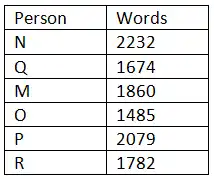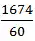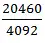# gkrecall

We brings you daily Current affairs, daily Current Affairs Quiz, weekly Current Affairs, weekly Current Affairs Quiz to enhance your preparation for upcoming exams. We also provide articles related to quant, reasoning, English, ssc etc .

## QUANT SET 28 FOR RRB PO & CLERK PRE-2020

RRB Clerk and RRB PO Prelims exam are going to be held in the upcoming months. We have already provided you with the PDFs of many topics of Quantitative Aptitude like Simplification/Approximation, Number Series,datainterpretation.important Arithmetic questions to prepare for RRB Clerk and PO Pre exam. Practicing these questions will help you to know about the level of the questions. To increase your speed and accuracy, enhance your calculations.

Web

### START QUIZ

1.42 liters of mixture contains milk and water in the ratio of 5 : 2. 50% of the mixture is taken out and replaced by water. If this process is repeated three times, the mixture contains x liter of milk. Find the value of x.

A3.75 liters
B3.25 liters
C3.5 liters
D2.5 liters
E2.75 liters

2.Two trains A and B whose speed is 100 km/h and X km/h respectively. If their average speed is 30% higher than the train B’s speed. Find out the approx. speed of train B.

A45 km/h
B80 km/h
C65 km/h
D54 km/h
ENone of the above

3.A shopkeeper bought an item of Rs. 500 and sells it at 20% profit but one customer lends it to him on selling price for 4 year at simple rate of interest is 5% per annum. How much profit percentage is earned by shopkeeper if interest is also considered as profit ?

A40%
B48%
C44%
D46%
ENone of these

4.Salary of a salesman is Rs. 7000 per month. Additionally, he is given a 5% commission on his sale and a 2% bonus on sale after Rs. 50000. If he earns Rs. 13700 in a month, find out the total sale.

A150000
B140000
C130000
D120000
E110000

5.A boat running upstream takes 9 hours 36 minutes to cover a certain distance, while it takes 7 hours 12 minutes to cover the same distance running downstream. What is the ratio between the speed of current to the speed of boat in still water ?

A5 : 2
B6 : 1
C5 : 3
D7 : 1
E4 : 1

6.Profit earned on selling an article at Rs 1200 is equals to the lost occurred by selling it on Rs.900.Find the cost price of an article.

ARs 1000
BRs 1050
CRs 1100
DRs 850
ENone of these

(7-10)Directions : Study the following information carefully and answer the following questions.

Six employees i.e. M, N, O, P, Q and R work for an organization. Each one of them types different number of words in an hour. Number of words typed by N in an hour are 20% more than that of M. Number of words typed by P in an hour are 40% more than that of O. Number of words typed by Q in an hour are 10% less than that of M. Number of words typed by R in an hour are equal to average words typed by O and P together in an hour. Total number of words typed by M and O in an hour are 3345. Number of words typed by M in an hour are 375 more than that of O.

7.Number of words typed by R in an hour are:

A1782
B1782
C1762
D1762
E1777

8.Approximately how much words are typed by Q in a minute?

A20
B23
C28
D30
E25

9.In how much time will M and N will be able to type 20460 words while working together?

A7 hours
B10 hours
C4 hours
D6 hours
E5 hours

10.Number of words typed by N in 3 hours is how much more than the number of words typed by R in 2 hour?

A3313
B3213
C3422
D3132
E3412

SOLUTION:

1.Final = Initial (1 – x/c)n
Where x = quantity replaced, c = total capacity and n = number of times process is done
According to the question,
Milk in the final capacity = 30(1 – 1/2)3
⇒ Milk in the final capacity = 30 × 1/8 = 3.75 litres

2.Average speed = 130% of speed of 2nd train
⇒ 2 × 100 × X/(100 + X) = (130/100) × X
⇒ 2 × 100 × 10 × X = (100 + X) × 13X
⇒ 2000X = 1300X + 13X2
⇒ 13X2 = 700X
⇒ X = 700/13 = 53.85 km/h ≈ 54 km/h
∴ The speed of 2nd train is 54 km/h.

3.Selling price = 120 × 500/100 = 600
Simple interest = (600 × 4 × 5)/100 = 120
Profit by selling the product = selling price - cost price = 600 - 500 = 100
Total profit earn by shopkeeper = 100 + 120 = 220
Profit percentage = (220/500) × 100 = 44%
∴ Profit percentage earned by shopkeeper = 44%

4.Total Commission = Total earning of a month – Salary of a month
⇒ Total Commission = 13700 – 7000 = 6700
Let, the total sale be of x rupees.
⇒ Total commission = Commission + bonus
⇒ 6700 = [x ×(5/100)] + [(x – 50000)×(2/100)]
⇒ 6700 = (x/20) + (x – 50000) /50
⇒ 6700 × 100 = 5(x) + 2(x – 50000)
⇒ 670000 = 5x + 2x – 100000 =
⇒ 770000 = 7x
⇒ x = 770000/7 = 110000.
∴ The total sale by a salesman is Rs. 110000.

5.Ratio of time = 576 : 432 = 4 : 3
⇒ Ratio of speed = 3 : 4
Let the upstream speed be 3R and downstream speed be 4R
From here speed of boat = (4R + 3R)/2 = 7R/2
And speed of stream = (4R – 3R)/2 = R/2
∴ Required ratio will be = (7R/2)/(R/2) = 7 : 1

6.Let the CP be x.
ATQ,
(1200-x)=(x-900)
2x=2100
X=Rs 1050

7.Solution:Let the number of words typed by M and O in an hour are m and o respectively.
We have,
m + o = 3345
m – o = 375
By solving both these equations, we have,
m = 1860, o = 1485
Number of words typed by M in an hour = 1860
Number of words typed by N in an hour = 120% of 1860 = 2232
Number of words typed by O in an hour = 1485
Number of words typed by P in an hour = 140% of 1485 = 2079
Number of words typed by Q in an hour = 90% of 1860 = 1674
Number of words typed by R in an hour = (1485 + 2079)/2 = 1782

8.Solution:Required answer ==28 (approx)

9.Total number of words typed by M and N together in an hour = 1860 + 2232 = 4092
Required time == 5